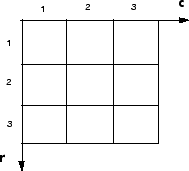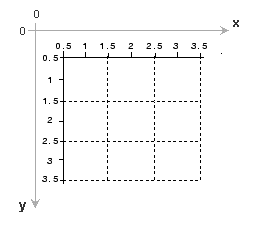## Expressing Image Locations

See published MATLAB coord.m

### Pixel Indices

Often, the most convenient method for expressing locations in an image is to use pixel indices. The image is treated as a grid of discrete elements, ordered from top to bottom and left to right, as illustrated by the following figure.

Pixel IndicesFor pixel indices, the row increases downward, while the column increases to the right. Pixel indices are integer values, and range from 1 to the length of the row or column.

There is a one-to-one correspondence between pixel indices and subscripts for the first two matrix dimensions in MATLAB. For example, the data for the pixel in the fifth row, second column is stored in the matrix element (5,2). You use normal MATLAB matrix subscripting to access values of individual pixels. For example, the MATLAB code

`I(2,15)`

returns the value of the pixel at row 2, column 15 of the image I. Similarly, the MATLAB code

`RGB(2,15,:) `

returns the R, G, B values of the pixel at row 2, column 15 of the image RGB.

The correspondence between pixel indices and subscripts for the first two matrix dimensions in MATLAB makes the relationship between an image's data matrix and the way the image is displayed easy to understand.

### Spatial Coordinates

Another method for expressing locations in an image is to use a system of continuously varying coordinates rather than discrete indices. This lets you consider an image as covering a square patch, for example. In a spatial coordinate system like this, locations in an image are positions on a plane, and they are described in terms of x and y (not row and column as in the pixel indexing system). From this Cartesian perspective, an (x,y) location such as (3.2,5.3) is meaningful, and is distinct from pixel (5,3).

#### Intrinsic Coordinates

By default, the toolbox uses a spatial coordinate system for an image that corresponds to the image's pixel indices. It's called the intrinsic coordinate system and is illustrated in the following figure. Notice that y increases downward, because this orientation is consistent with the way in which digital images are typically viewed.

Spatial Coordinate SystemThe intrinsic coordinates (x,y) of the center point of any pixel are identical to the column and row indices for that pixel. For example, the center point of the pixel in row 5, column 3 has spatial coordinates x = 3, y = 5. This correspondence simplifies many toolbox functions considerably. Be aware, however, that the order of coordinate specification (3,5) is reversed in intrinsic coordinates relative to pixel indices (5,3).

Several functions primarily work with spatial coordinates rather than pixel indices, but as long as you are using the default spatial coordinate system (intrinsic coordinates), you can specify locations in terms of their columns (x) and rows (y).

When looking at the intrinsic coordinate system, it's easy to see that the upper left corner of the image is located at (0.5,0.5) and the lower right corner of the image is located at (numCols + 0.5, numRows + 0.5), where numCols and numRows are the number of rows and columns in the image. In contrast, the upper left pixel is pixel (1,1) and the lower right pixel is pixel (numRows, numCols). The center of the upper left pixel is (1.0, 1.0) and the center of the lower right pixel is (numCols, numRows). In fact, the center coordinates of every pixel are integer valued. The center of the pixel with indices (r, c) — where r and c are integers